Worksheets and No Prep Teaching Resources Math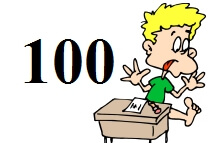Counting to a Hundred Printables, Worksheets, and Lessons

Hundreds Chart

Doing 100 Things - Chart for Bulletin Board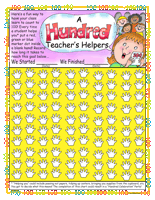"A Hundred Teacher's Helpers" Basics Chart

Counting to 100 Days of School Chart for Bulletin Board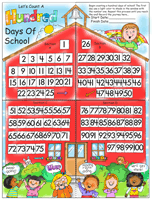"Let's Count A Hundred Days Of School" Jumbo Chart

Ordering Numbers
Numbers: 0-10
Fill in the missing numbers (Just before, after, and between)
Greater than, less than, and equal to (circle the words)
Circle the larger number
Circle the smaller number
Order 3 numbers (from smallest to largest)
Order 3 numbers (from largest to smallest)
Order 4 numbers
Ordering Mixed Review

Numbers: 11-20
Fill in the missing numbers (Just before, after, and between)
Greater than, less than, and equal to (circle the words)
Circle the larger number
Circle the smaller number
Order 3 numbers (from smallest to largest)
Order 3 numbers (from largest to smallest)
Order 4 numbers
Ordering Mixed Review

Numbers: 0 to 20
Fill in the missing numbers (Just before, after, and between)
Greater than, less than, and equal to (circle the words)
Circle the larger number
Circle the smaller number
Order 3 numbers (from smallest to largest)
Order 3 numbers (from largest to smallest)
Order 4 numbers
Ordering Mixed Review

Numbers: 0 to 50
Fill in the missing numbers (Just before, after, and between)
Greater than, less than, and equal to (circle the words)
Circle the larger number
Circle the smaller number
Order 3 numbers (from smallest to largest)
Order 3 numbers (from largest to smallest)
Order 4 numbers
Ordering Mixed Review

Numbers: 0 to 99
Fill in the missing numbers (Just before, after, and between)
Greater than, less than, and equal to (circle the words)
Circle the larger number
Circle the smaller number
Order 3 numbers (from smallest to largest)
Order 3 numbers (from largest to smallest)
Order 4 numbers
Ordering Mixed Review

Place Value to 99: Ones and Tens
Numbers 11 to 49
Graphics: count and write the number of ones and tens
Given a number: write the number of ones and tens
Given ones and tens: write the number
Fill in the missing number
Circle the larger number
Circle the smaller number

Numbers 51 to 99
Graphics: count and write the number of ones and tens
Given a number: write the number of ones and tens
Given ones and tens: write the number
Fill in the missing number
Circle the larger number
Circle the smaller number

Numbers 1 to 99
Graphics: count and write the number of ones and tens
Given a number: write the number of ones and tens
Given ones and tens: write the number
Fill in the missing number
Circle the larger number
Circle the smaller number

Review
Place Value to 99: Ones and Tens Review

100 Days of School

Daily 100 Days Chart for the Classroom or Bulletin Board - New Page for Each Day - 100 Total Pages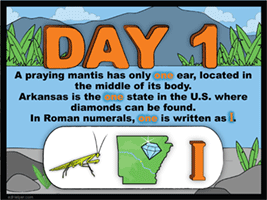100 Days - Day 1 through Day 10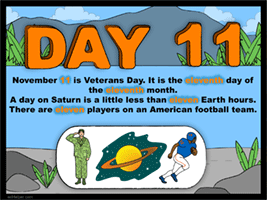100 Days - Day 11 through Day 20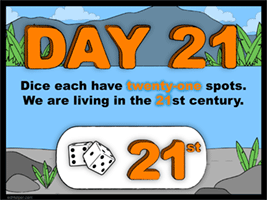100 Days - Day 21 through Day 30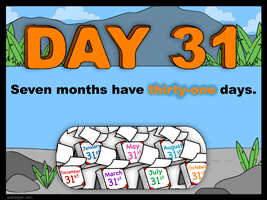100 Days - Day 31 through Day 40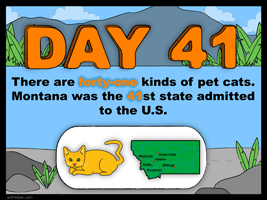100 Days - Day 41 through Day 50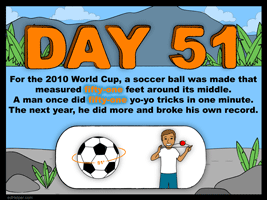100 Days - Day 51 through Day 60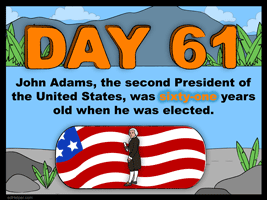100 Days - Day 61 through Day 70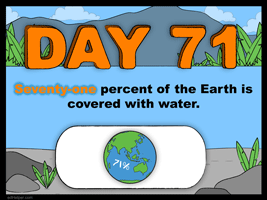100 Days - Day 71 through Day 80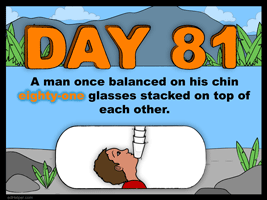100 Days - Day 81 through Day 90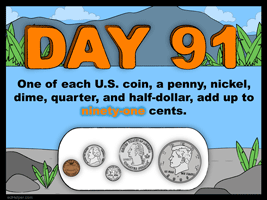100 Days - Day 91 through Day 100

Skip Counting Printables
 Counting by twos Counting by threes Counting by fours Counting by fives Counting by sixes Counting by sevens Counting by eights Counting by nines Counting by tens Skip Counting

Have a suggestion or would like to leave feedback?